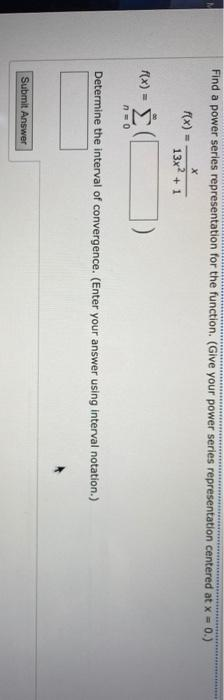### Create an Account

Home / Questions / Find a power series representation for the function. (Give your power series representatio...

# Find a power series representation for the function. (Give your power series representation centered at x = 0. х f(x) 13x2 + 1 f(x) = Σ į n=0 Determine the interval of convergence. (Enter your answer

Find a power series representation for the function. (Give your power series representation centered at x = 0. х f(x) 13x2 + 1 f(x) = Σ į n=0 Determine the interval of convergence. (Enter your answer using interval notation.) Submit AnswerJun 02 2021 View more View LessSubscribe To Get Solution